# Test Prep Plan - Take a practice test

Take this practice test to check your existing knowledge of the course material. We'll review your answers and create a Test Prep Plan for you based on your results.
How Test Prep Plans work
1
2Based on your results, we'll create a customized Test Prep Plan just for you!
3Study smarter
Study more effectively: skip concepts you already know and focus on what you still need to learn.

# NES Math: Circles & Arc of a Circle Chapter Exam

Exam Instructions:

Choose your answers to the questions and click 'Next' to see the next set of questions. You can skip questions if you would like and come back to them later with the yellow "Go To First Skipped Question" button. When you have completed the practice exam, a green submit button will appear. Click it to see your results. Good luck!

### Page 1

#### Question 3 3. In the picture below, if AB is 7, BC is 19 and AD is 8, what is the length of DE?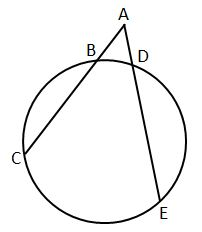#### Question 5 5. In the picture below, if arc AB is 82 degrees, what is the measure of angle BAC?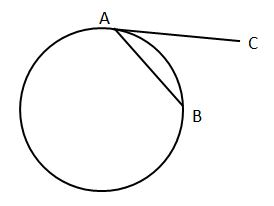### Page 2

#### Question 6 6. In the picture below, if AC is 14 and AB is 10, what is the length of AD?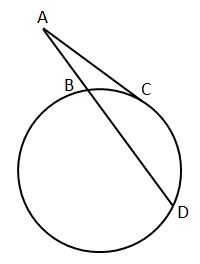#### Question 7 7. If arc AB is 72 degrees, what is the measure of angle ACB?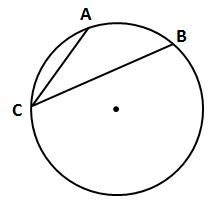#### Question 9 9. If arc AC is 106 degrees and arc BC is 82 degrees, what is the measure of angle ACB?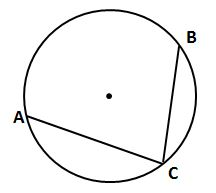### Page 3

#### Question 11 11. In the picture below, if major arc BC is 258 degrees and minor arc BC is 102 degrees, what is the measure of angle BAC?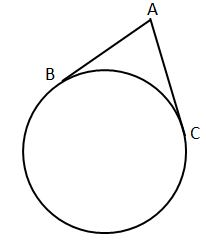#### Question 12 12. In the pictured circle, the measure of angle ACB is 22 degrees and the measure of angle BXD is 120 degrees. What is the measure of angle CAD?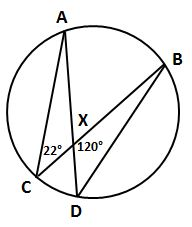#### Question 13 13. If the measure of central angle AOB is 62 degrees, what is the measure of inscribed angle ACB?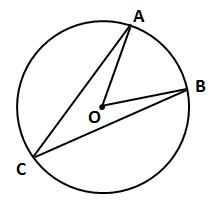#### Question 14 14. If angle OAB is 45 degrees, what is the measure of angle AOB?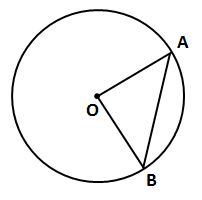#### Question 15 15. Which line is a tangent to the pictured circle?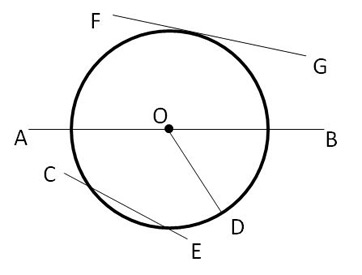### Page 4

#### Question 16 16. In the picture below, if AB is 12, AC is 24 and AD is 10, what is the length of AE?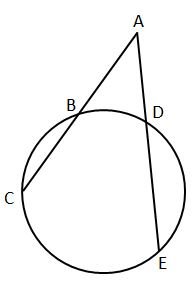#### Question 18 18. In the picture below, if arc AC is 146 degrees and arc BD is 32 degrees, what is the measure of angle AEC?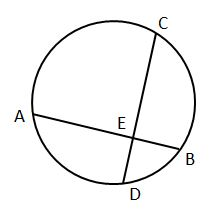### Page 5

#### Question 24 24. In the picture below, if arc BD is 168 degrees and arc BC is 70 degrees, what is the measure of angle BAD?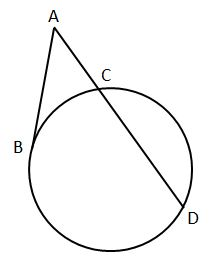#### Question 25 25. In the picture below, if AE is 10, AB is 14 and ED is 16, what is the length of CE?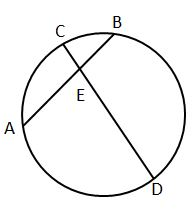### Page 6

#### Question 26 26. If angle ACB is 47 degrees, what is the measure of angle AOB?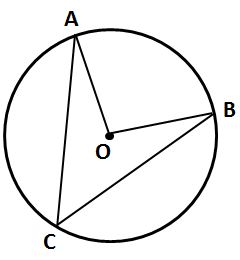#### Question 30 30. If angle ACB is 34 degrees, what is the measure of angle ADB?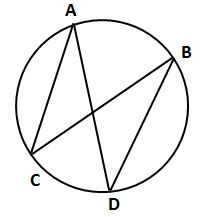#### NES Math: Circles & Arc of a Circle Chapter Exam Instructions

Choose your answers to the questions and click 'Next' to see the next set of questions. You can skip questions if you would like and come back to them later with the yellow "Go To First Skipped Question" button. When you have completed the practice exam, a green submit button will appear. Click it to see your results. Good luck!

Support